# Bihar Board Class 12th Physics Solutions Chapter 5 Magnetism and Matter

Bihar Board Class 12 Physics Solutions Chapter 5 Magnetism and Matter Textbook Questions and Answers, Additional Important Questions, Notes.

## BSEB Bihar Board Class 12 Physics Chapter 5 Magnetism and Matter

### Bihar Board Class 12 Physics Magnetism and Matter Textbook Questions and Answers

Question 1.
Answer the following questions regarding earth’s magnetism:
(a) A vector needs three quantities for its specification. Name the three independent quantities conventionally used to specify the earth’s magnetic field.
(b) The angle of dip at a location in southern India is about 18°. Would you expect a greater or smaller dip angle in Britain?
(c) If you made a map of magnetic field lines at Melbourne in Australia, would the lines seen to go into the ground or come out of the ground?
(d) In which direction would a compass free to move in the vertical plane point to, if located right on the geomagnetic north or south pole?
(e) The earth’s field, it is claimed, roughly, approximates the field due to a dipole of magnetic moment 8 x 1022 JT-11 located at its centre. Check the order of magnitude of this number in some way.
(f) Geologists claim that besides the main magnetic N – S poles, there are several local poles on the earth’s surface oriented in different directions. How is such a thing possible at all?
(a) The three independent quantities conventionally used to specify the earth’s magnetic field are magnetic declinatiion (θ), horizontal component of earth’s magnetic field (BH) and magnetic dip (δ).
(b) Yes, Great Britain will have a greater dip angle because it is located closer to the magnetic North Pole. The value of magnetic dip there is about 70°.
(c) The magnetic lines of force will seem to come out of ground at
Melbourne because it is situated in Southern hemisphere where north pole of earth’s magnetic field lies.
(d) At the magnetic poles earth’s magnetic field is exactly vertical and as the compass needle is free to rotate in horizontal plane, so the compass may point in any direction there.
(e) The magnetic field at a point on equatorial line of magnetic dipole (assuming earth has magnetic dipole) is given by
might be the ‘battery’ (i.e., the source of energy) to sustain these currents?
(d) The earth may have even reversed the directin of its field several times during its history of 4 to 5 billion years. How can geologists know about the earth’s field in such distant past?
(e) The earth’s field departs from its dipole shape substantially at large distances (greater than about 30,000 km). What agencies may be responsible for this distortion?
B = $$\frac{\mu_{0}}{4 \pi} \cdot \cdot \frac{\mathrm{M}}{\mathrm{r}^{3}}$$
Taking M =8 x 1022 JT-1 and r = 6.4 x 106 m = radius of earth.
∴ B = $$\frac{4 \pi \times 10^{-7}}{4 \pi} \times \frac{8 \times 10^{22}}{\left(6.4 \times 10^{6}\right)^{3}}$$
= 0.3 x 10-4T = 0.3G.
The above value is almost same as the earth’s magnetic field. This checks the magnitude of the dipole moment.
(f) Yes, this is possible. The earth’s magnetic field is only approximately a diple field. Thus, local N – S poles may exist oriented in different directions. This is possible due to deposits of magnetised materials.Question 2.
(a) The earth’s magnetic field varies from point to point in space. Does it also change with time? If so, on what time scale does it change
appreciably?
(b) The earth’s core is known to contain iron. Yet geologists do not regard this as a source of’the earth’s magnetism. Why?
(c) The charged currents in the outer conducting regions of the earth’s core are thought to be responsible for earth’s magnetism. What might be the ‘battery’ (i.e., the source of energy) to sustain these currents?
(d) The earth may have even reversed the directin of its field several times during its history of 4to 5 billion years. How can geologists know about the earth’s field in such distant past?
(e) The earth’s field departs from its dipole shape substantially at large distances (greater than about 30,000 km). What agencies may be responsible for this distortion?
(f) Interstellar space has an extremely weak magnetic field of the order of 10-12 T. Can such a weak field be of any significant consequence? Explain.
[Note : Exercise 5.2 is meant mainly to arouse your curiosity. Answers to some questions above are’ tentative or unknown. Brief answers wherever possible are given at the end. For details, you should consult a good text on geomagnetism.]
(a) Yes, earth’s magnetic field changes with time for say, annual changes, daily changes, secular changes with period of about 960 years. Magnetic storms are considered as irregular changes. The time for an appreciable change is roughly few hundred years.
(b) Molten iron cannot retain magnetism because it is above its curie temperature.
(c) Radioactivity in the interior of earth but it is not certain.
(d) Earth’s magnetic field gets weakly recorded in certain rocks during solidification. Analysis of this rock magnetism gives us idea about geomagnetic history.
(e) If the distance is large, the magnetic field gets modified by the field produced by motion of ions in earth’s ionosphere.
(f) Tire deflection of a charged particle moving in a magnetic field is given by
Be v = $$\frac{\mathrm{mv}^{2}}{\mathrm{r}}$$ or r = $$\frac{\mathrm{mv}}{\mathrm{Be}}$$
If B is low, r is high, i.e. radius of curvature of path is very large. Thus, if distance is very large for eg. is space, the charged particle is hardly noticeable.

Question 3.
A short bar magnet placed with its axis at 30° with a uniform external magnetic field of 0.25 T experience a torque of magnitude equal to 4.5 x 10 J. What is the magnitude of magnetic moment of the magnet ?
Here, θ = 30°
B = uniform external magnetic field = 0.25 T
τ = torque = 4.5 x 10-2 ]
M = magnitude of magnetic moment of magnet = ? We know thatQuestion 4.
A short bar magnet of magnetic moment m = 0.32 JT-1 is placed in a uniform magnetic field of 0.15 T. If the bar is free to rotate in the plane of the field, which orientation would correspond to its (i) stable and (ii) unstable equilibrium? What is the potential energy of the magifc in each case?
Here m = magnetic moment of bar magnet = 0.32 J T-1
B = uniform magnetic field = 0.15 T
(i) When $$\overrightarrow{\mathrm{m}}$$ is parallel to $$\overrightarrow{\mathrm{B}}$$ , the magnet is in stable equilibrium,
∴ θ = 0°
∴ P.E. in this case is given by
U = $$\overrightarrow{\mathrm{m}}$$ . $$\overrightarrow{\mathrm{B}}$$ = – m B cos θ
= -0.32 x 0.15 x 1 =-0.048 J.

(ii)When m is anti  parallel to B , the magnet will be in unstable equilibrium,
.’. θ = 180°
Thus Potential energy in this case is given by
U = – $$\overrightarrow{\mathrm{m}}$$ . $$\overrightarrow{\mathrm{B}}$$ = – mB cos 180°
= – 0.32 x 0.15 x (-1) = + 0.048 J.

Question 5.
A closely wound solenoid of 800 turns and area of cross section 2.5 x 10-4 m2 carries a current of 3.0 A. Explain the sense in which the solenoid acts like a bar magnet. What is its associated magnetic moment?
Here, N = number of turns = 800.
A = area of cross-section of solenoid = 2.5 x 10-4 m2.
I = current in the solenoid = 3.0 A.
Let M = associated magnetic moment = ?
We know that M for a current carrying solenoid is given by:
M = NIA
= 800 x 3.0 x 2.5 x 10-4
= 60 x 10-2 Am2
= 0.60 Am2 = 0.60 JT-1
Which acts along the axis of the solenoid in the direction related to the sense of flow of current according to right handed screw rule.Question 6.
If the solenoid in exercise 5.5 is free to turn about the vertical direction and a uniform horizontal magnetic field of 0.25 T is applied, what is the magnitude of torque on the solenoid when its axis makes an angle of 30° with the direction of applied field?
Here B = uniform horizontal magnetic field = 0.25 T
θ = angle made by $$\overrightarrow{\mathrm{m}}$$ (i.e., the axis of solenoid with $$\overrightarrow{\mathrm{B}}$$ = 30°
$$\overrightarrow{\mathrm{m}}$$ = 0.60 Am2
τ = magnitude of Torque on the solenoid = ?We know that x is given by
x = MB sin θ
= 0.60 x 0.25 x sin 30°
= 0.6 x 0.25 x $$\frac {1}{2}$$
= 0.075 Nm.

Question 7.
A bar magnet of magnetic moment 1.5 JT-1 lies aligned with the direction of a uniform magnetic field of 0.22 T.
(a) What is the amount of work required by an external torque to turn the magnet so as to align its magnetic moment,
(i) normal to the field direction
(ii) opposite to the field direction?
(b) What is the torque on the magnet in cases (i) and (ii)?
Here, M = magnetic moment of bar magnet =1.5 JT-1
B = uniform magnetic field = 0.22 T.
θ 1= 0 as the dipole is aligned with “g .
(a) (i) When the bar magnet is displaced to perpendicular position, then θ 2 = 90°
If W = work done so as to align $$\overrightarrow{\mathrm{m}}$$ normal to $$\overrightarrow{\mathrm{B}}$$ then using the relation,
W = MB (cos θ 1 – cos θ 2), we get
W = 1.5 x 0.22 (cos 0 – cos 90°)
= 1.5 x 0.22(1 – 0)
= 0.33 J.

(ii) When the magnet is displaced so as to align $$\overrightarrow{\mathrm{M}}$$ opposite to $$\overrightarrow{\mathrm{B}}$$ ,then
θ 2= 180°
τ2 = MB sin θ 2
= 1.5 x 0.22 x sin 180°
= 0.33 x (0) = 0

If W’ be the work done in this case, then
W’ = MB (cos θ 1 – cos θ 2)
= 1.5 x 0.22 (cos.θ – cos 180°)
= 0.33 [1 – (-1)]
= 0.33 x 2 =0.66 J.

(b) (i) Let τ1 be the torque on the magnet when it is aligned ⊥ ar to $$\overrightarrow{\mathrm{B}}$$ , then using the relation,
τ = MB sin θ, We get
τ1 = MB sin θ1
τ1 = 1.5 x 0.22 x sin 90° (θ1 = 90°)
= 0.33 x 1 = 0.33 Nm-2.

(ii) Let τ2 be the torque in case (ii)
Here, θ2 = 180°
∴ τ2 = MB sin θ2
= 1.5 x 0.22 x sin 180°
= 0.33 x (0) = 0.

Question 8.
A closely wound solenoid of 2000 turns and area of crosssection 1.6 x 10-4 m2, carrying a current of 4.0 A, is suspended through its centre allowing it to turn in a horizontal plane.
(a) What is the magnetic moment associated with the solenoid?
(b) What is the force and torque on the solenoid if a uniform horizontal magnetic field of 7.5 x 10-2 T is set up at an angle of 30° with the axis of the solenoid?
Here, N = Number of turns in the solenoid = 2000
A = area of cross-section of solenoid = 1.6 x 10-4 m2
I = current in the solenoid = 4.0 A
(a) Let M = magnetic moment of the solenoid.
∴ Using the relation M = NLA, we get
M = 2000 x 4.0 x 1.6 x 10-4
= 1.28 JT-1.
The direction of $$\overrightarrow{\mathrm{M}}$$ is along the axis of the solenoid in the direction related to the sense of current according to right-handed screw rule.(b) Here θ = 30°
$$\overrightarrow{\mathrm{B}}$$ = 7.5 x 10-2 T
Let F = force on the solenoid = ?
τ = torque on the solenoid = ?
Tire solenoid behaves as a barmagnet placed in a uniform magnetic field, so the force is
∴ F = m $$\overrightarrow{\mathrm{B}}$$ + (- m $$\overrightarrow{\mathrm{B}}$$ ) = 0.
where m = pole strength of the magnet.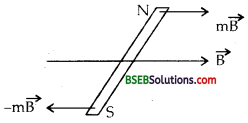Using the relation, t = MB sin θ, we get
τ = 1.28 x 7.5 x 10-2 x sin 30°
= 1.28 x 7.5 x 10-2 x $$\frac {1}{2}$$ = 0.048 J.
The direction of the torque is such that it tends to align the axis of the solenoid (i.e,, magnetic moment vector $$\overrightarrow{\mathrm{M}}$$ ) along $$\overrightarrow{\mathrm{B}}$$.

Question 9.
A circular coil of 16 turns and radius 10 cm carrying a current of 0.75 A rests with its plane normal to an external field of magnitude 5.0 x 10-2 T. The coil is free to turn about an axis in its plane perpendicular to the field direction. When the coil is turned slightly and released, it oscillates about its stable equilibrium with a frequency of 2.0 s-1. What is the moment of inertia of the coil about its axis of rotation ?
Here, N = 16, r = radius of coil = 10 cm = 0.10 m
I = current in the coil = 0.75 A.
BH = external.magnetic field = 5.0 x 10-2 T
v = frequency of oscillation of the coil = 2.0 s-1.
I = Moment of Inertia of the coil = ?
Let A = area of cross-section of the coil = πr2 = n (0.01)2 = 104 πm2.
If M be the magnetic moment of the coil, then
M = NIA = 16 x 0.75 x 10-4 it Am2.
Also let T be the time period of oscillation of the coil.
∴ T = $$\frac {1}{υ}$$ = $$\frac {1}{2}$$ = 0.5 s
Also we know that .Question 10.
A magnetic needle free to rotate in a vertical plane parallel to the magnetic meridian has its north tip pointing down at 22° with the horizontal. The horizontal component of the earth’s magnetic field at the place is known to be 0.35 G. Determine the magnitude of the earth’s magnetic field at the place.
Here
BH = horizontal component of earth’s magnetic field = 0.35 G.
ρ = angle of dip = 22°
Let B be the magnitude of earth’s magnetic field at the place = ?
We know that BH = B cos δ
B = $$\frac{\mathrm{B}_{\mathrm{H}}}{\cos \delta}=\frac{0.35}{\cos 22^{\circ}}=\frac{0.35}{0.9272}$$
= 0.38 G.

Question 11.
At a certain location in Africa, a compass points 12° west of the geographic north. The north tip of the magnetic needle of a dip circle placed in the plane of magnetic meridian points 60° above the horizontal. The horizontal component of the earth’s field is measured to be 0.16 G. Specify the direction and magnitude of the earth’s field at the location.
Here, 0 = angle of declination = 12°
δ = angle of dip = 60°
BH = horizontal component of earth’s magnetic field = 0.16 G.Let $$\overrightarrow{\mathrm{B}}$$ = earth’s magnetic field at that location = ?
Using the relation, BH = B cos δ, we get the magnitude of $$\overrightarrow{\mathrm{B}}$$ given by,
B = $$\frac{\mathrm{B}_{\mathrm{H}}}{\cos \delta}=\frac{0.16}{\cos 60^{\circ}}=\frac{0.16}{\left(\frac{1}{2}\right.)}$$
= 0.6 x 2 = 0.32 G
or B = 0.32 x 10-4 (∴ 1 G = 10-4 T)

Direction of $$\overrightarrow{\mathrm{B}}$$ The earth’s field lies in a vertical plane 12c west of geographic meridian at an angle of 60c above the horizontal line.

Question 12.
A short bar magnet has a magnetic moment of 0.48 J T-1. Give the direction and magnitude of the magnetic field produced by the magnet at a distance of 10 cm from the centre of the magnet on (i) the axis (ii) the equatorial lines (normal bisector) of the magnet.
Here, Mp magnetic moment of bar magnet = 0.48 J T-1.
r = distance of’the point from the centre of the magnet = 10 cm = 0.1 m.

(i) When the point lies on the axis :
Let B1 be the magnetic field at P.
∴ B1 = $$\frac{\mu_{0}}{4 \pi} \cdot \frac{2 M}{r^{3}}$$ = 10-7 x $$\frac{2 \times 0.48}{(0.1)^{3}}$$
= 0.96 x 10-4 T along S.N or NP
= 0.96 G along S-N direction.(ii) Let $$\overrightarrow{\mathrm{B}_{\mathrm{2}}}$$ be the magnetic field at point P on the equitorial line.Question 13.
A short bar magnet placed in a horizontal plane has its axis aligned along the magnetic north-south direction. Null points are found on the axis of the magnet at 14 cm from the centre of the magnet. The earth’s magnetic field at the place is 0.36 G and the angle of dip is zero. What is the total magnetic field on the normal bisector of the magnet at the same distance as the null point (i.e., 14 cm) from the centre of the magnet? (At null points, field due to a magnet is equal and opposite to the horizontal component of earth’s magnetic field.)
Here, B = Earths total magnetic field = 0.36 G. = 0.36 x 10-4 T.
δ = angle of dip = 0
r = distance of the null points on the axis of magnet = 0.14 m.
Let B’ be the fieLd due to the magnet at a point on the axial line.
∴ B’ = $$\frac{\mu_{0}}{4 \pi} \cdot \frac{2 \mathrm{M}}{\mathrm{r}^{3}}$$ ……..(i)
Also we know that at null points
B’ = BH ………(ii)
and BH = B cos δ = B cos 0 = B = 0.36 x 10-4T ………(iii)
∴ From (1), (ii) and (iii), we getLet Beqt be the magnetic field due to the magnet at a point on the equitorial line at a distance 0.14 m from its centre.Let Bt be the total magnetic field at point on the normal bisector at 0.14 m from the centre of magnet. Then, Bt = Bmagnet + Bearth
or Bt = Beqt + BH
= 0.18+ 0.36 = 0.54 G
in the direction of earth’s field.Question 14.
If the bar magnet in Exercise 5.13 is turned around by 180°, where will the new null-points be located?
Where the magnet is turned through 180° i.e. reversed i.e., north pole is pointing north, the neutral points will lie on the equitorial line of the magnet. If r be the distance of the neutral points on the equitorial line, then the magnetic field Beqt is given by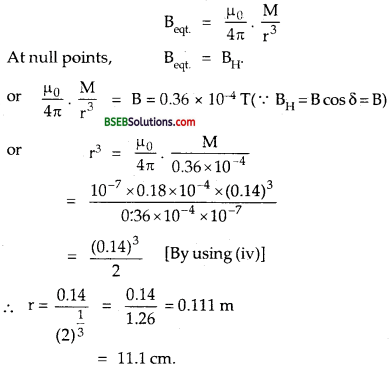Question 15.
A short bar magnet of magnetic moment 5.25 x 10-2 J I-1 is placed with its axis perpendicular to the earth’s field direction. At what distance from the centre of the magnet, the resultant field is inclined at 45° with earth’s field on (a) its normal bisector and (b) its axis. Magnitude of the earth’s field at the place is given to be 0.42 G. Ignore the length of the magnet in comparison to the distances involved.
Here, M = 5.25 x 102 JT-1
BH = 0.42 G = 0.42 x 10-4 T.

(a) On its normal bisectr :
Let r be the distance of point Pon the equitorial line at which the resultant magnetic field $$\overrightarrow{\mathrm{B}}$$ is inclined at 45°with the earth’s field BH. For it to happen, Beqt must be equal to BH.
i.e Beqt = BH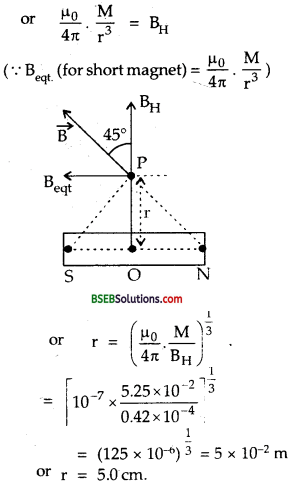(b) On its axis :
Let r be the distance of the point Pon the axis of the magnet where the resultant field is inclined at 45 with the earth’s magnetic field.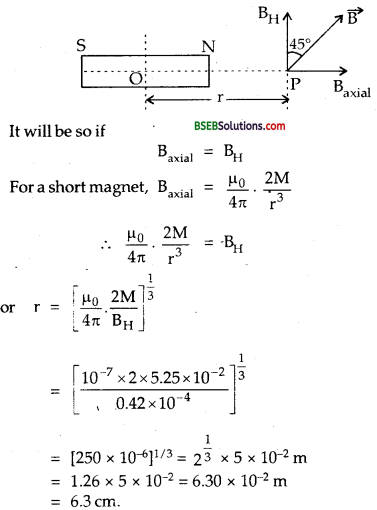Question 16.
(a) Why does a paramagnetic sample display greater magnetisation (for the same magnetising field) when cooled?
(b) Why is diamagnetism, in contrast, almost independent of temperature?
(c) If a toroid uses bismuth for its core, will the field in the core be (slightly) greater or (slightly) less than when the core is empty?
(d) Is the permeability of a ferromagnetic material independent of the magnetic field? If not, is it more for lower or higher fields?
(e) Magnetic field lines are always nearly normal to the surface of a ferromagnet at every point. (This fact is analogous to the static electric field lines being normal to the surface of a conductor at every point.) Why?
(f) Would the maximum possible magnetisation of a paramagnetic sample be of the same order of magnitude as the magnetisation of a ferromagnet?
(a) On cooling, the tendency of thermal agitations to disrupt the alignment of magnetic dipoles decreases in case of paramagnetic materials. Thus, they display greater amount of magnetism.
(b) On placing a sample of diamagnetic material in magnetic field., the magnetisation (induced dipolement) is opposite to direction of magnetising field. Thus, it is not affected by temperature.
(c) The field in the core will be slightly less than when the core is emptly as Bismuth is diamagnetic.
(d) No; because permeability of ferromagnetic material is dependent on applied magnetic field. Graph for B and H is shown. B is large for smaller value of H, thus permeability μ (= B/H) is greater for lower fields.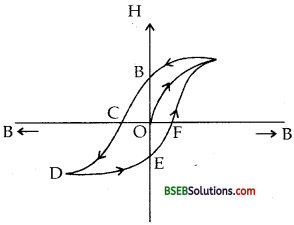(e) The field lines meet the material normally as μr »1.
(f) Yes. A paramagnetic substance sample with satured magnetism will have same order of magnetisation of a ferromagnet. However, a saturated magnetisation will require very high field. There will be a minor difference in the strength of the atomic dipoles of paramagnetic and ferromagnetic materials.

Question 17.
(a) Explain qualitatively on the basis of domain picture the irreversibility in the magnetisation curve of a ferromagnet.
(b) The hysteresis loop of a soft iron piece has a much smaller area than that of a Carbon Steel piece. If the material is to go through repeated cycles of magnetisation, which piece will dissipate greater heat energy?
(c) A system displaying a hysteresis loop such as a ferromagnet, is a device for storing memory? Explain the meaning of this statement.
(d) What kind of ferromagnetic material is used for coating magnetic tapes in a cassette player, or for building ‘memory stores’ in a modem computer?
(e) A certain region of space is to be shielded from magnetic fields. Suggest a method.
(a) In a ferromagnetic material, atoms form a very large number of small effective regions called domains. Each domain has a linear dimension ≈ 1000 Å. Within each domain, a special interaction called exchange coupling renders dipole moments of all atoms in a particular direction. Thus each domain is a strong magnet without any external magnetic field but the ferromagnetic substance does not behave as a magnet in the absence of external magnetic field as the magnetic moments of different domains are randomly oriented.
(b) The carbon steel piece will dissipate greater heat energy as heat lost per cycle is proportional to the area of the hysteresis loop.
(c) The ferromagnetic substance shows that it remains magnetised even after the removal of external magnetic field. It means that magnetism is stored as a memory in the ferromagnet. Thus, a system displaying hysteresis is a device for storing memory.
(d) Ceramics are used for coating magnetic tapes in cassette players or for building. Memory stores in modem computers. Ceramics are double oxides of barium and iron. Ceramics are also called ferrites.
(e) It is done by surrounding the region with soft iron rings. Magnetic field lines will be drawn into rings and enclosed space will be free of magnetic fields. But this shielding is only approximate, unlike the perfect electric shielding of a cavity in a conductor placed in an external electric field.Question 18.
A long straight horizontal cable carries a current of 2.5 A in the direction 10° south of west to 10° north of east. The magnetic meridian of the place happens to be 10° west of the geographic meridian. The earth’s magnetic field at the location is 0.33 G, and the angle of dip is zero. Locate the line of neutral points (ignore the thickness of the cable)? (At neutral points, magnetic field due to a current carrying cable is equal and opposite to the horizontal component of earth’s magnetic field.)
The magnetic n.s line is 10° west of geographical N.S. line straight horizontal cable XY is 10°N of east.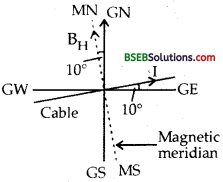Here, B = earth’s total magnetic field = 0.33 G = 0.33 x 10-4 T
I = 2.5 A = Current in the cable,
angle of dip, δ = 0
If BH be the horizontal component of earth’s field, then
BH = B cos δ = B cos0° = B = 0.33 G = 0.33 x 10-4T
At points below the cable, the direction of the magnetic field produced is same as that of BH, while at a point above the cable, the direction of the field produced is opposite to that of BH. So the neutral point lies above the cable.
Let r be the distance of the neutral points from the cable.
If Bmrg be the magnetic field due to the straight current carrying cable, then
Bmrg = $$\frac{\mu_{0}}{4 \pi} \cdot \frac{2 I}{r}$$
At netural pointsi.e., the neutral point lies on a line parallel to the cable and above it at 1.52 cm.

Question 19.
A telephone cable at a place has four long straight horizontal wires carrying a current of 1.0 A in the same direction east to west. The earth’s magnetic field at the place is 0.39 G, and the angle of dip is 35°. The magnetic declination is nearly zero. What are the resultant magnetic fields at points 4.0 cm below the cable?
Here, earth’s magnetic field, B = 0.39 G = 0.39 x 10-4 T
δ = angle of dip = 35°
θ = angle of magnetic declination = 0°.
n = number of wires = 4
1 = current = 1.0 A.
r = distance = 4 cm each = 0.04 m.
If Bv and Bu be the vertical and horizontal components of earth’s total field, then
BH = B cos δ = 0.39 cos 35° = 0.39 x 0.8192 = 0.3195 G
and Bv = B sin δ = 0.39 sin 35° = 0.39 x 0.5736 = 0.2237 G.
Let B1 be the field due to a single wire, then
B1 = $$\frac{\mu_{0}}{4 \pi} \cdot \frac{2 I}{r}$$

∴ If B’ be the total magnetic field produced by the four wires of the cable, then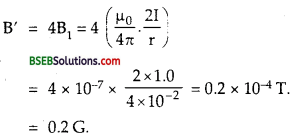At a point below cable :
At a point say Q, 4. cm below the wire, the horizontal component of earth’s magnetic field BH and the field due to current are in opposite direction, so the net horizontal field RH is given ‘by
RH = BH – B’ = 0.3195 – 0.2
= 0.1195 G = 0.12 G
Net vertical component, Rv = Bv = 0.22 37 G = 0.22 G.If R be the resultant magnetic field, thenDirection of $$\overrightarrow{\mathrm{R}}$$ :
Let 0 be the angle made by $$\overrightarrow{\mathrm{R}}$$ with the horizontal. Then,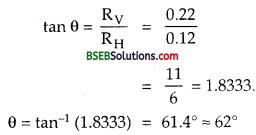Above the Cable :
Say at point. P, 4 cm above the wire, the horizontal component of earth’s field and the field due to the current are in the same direction.
∴ Resultant horizontal field RH is given by
RH = BH + B’ = 0.3195 + 0.2 = 0.5195 = 0.52 G
and resultant vertical field is
Rv = Bv = 0.22 G.If R’ be their resultant field above the cable, thenDirection of $$\overrightarrow{\mathrm{R}}$$ :
If θ1 be the angle made by $$\overrightarrow{\mathrm{R’}}$$ with RH, therQuestion 20.
A compass needle free to turn in a horizontal plane is placed at the centre of circular coil of 30 turns and radius 12cm. The coil is in a vertical plane making an angle of 45° with the magnetic meridian. When the current in the coil is 0.35 A, the needle points west to east.
(a) Determine the horizontal component of the earth’s magnetic field at the location.
(b) The current in the coil is reversed, and the coil is rotated about its vertical axis by an angle of 90° in the anticlockwise sense looking from above. Predict the direction of the needle. Take the magnetic declination at the places to be zero.
Here, n = no. of turns in the coil = 30
r = radius of circular coil = 12 m = 12 x 10-2 m.
I = current in the coil = 0.35 A.
(a) The horizontal component of the earth’s magnetic field (BH) acts along the magnetic N-S line. As the coil is placed in a vertical plane making angle 45° with the magnetic meridian, the magnetic field $$\overrightarrow{\mathrm{B}}$$ produced by the coil on passing current through it, will be along normal to the plane of the coil i.e., in a direction making angle of 45° with the WE direction. The compass needle placed at the centre of the coil points along W to E.From the figure, it follows that
BH = B sin 45° = B = $$\frac{1}{\sqrt{2}}$$ ……….(i)
The magnetic field produced at the centre of the coil is given by
B = $$\frac{\mu_{0}}{4 \pi} \cdot \frac{2 \pi \mathrm{I} \mathrm{I}}{\mathrm{r}}$$ ……..(ii)
From (i) and (ii),(b) The needle will reverse its original direction i.e., it will point east to west to the plane of coil makes an angle of 45° with the magnetic meridian on the other side, so the needle rotates.

Question 21.
A magnetic dipole is under the influence of two magnetic fields. The angle between the field directions is 60°, and one of the fields has a magnitude of 1.2 x 10-2 T. If the dipole comes to stable equilibrium at an angle of 15° with this field, what is the magnitude of the other field ?
The two fields $$\overrightarrow{\mathrm{B}_{\mathrm{1}}}$$ and $$\overrightarrow{\mathrm{B}_{\mathrm{2}}}$$ are shown in the figure here in which a magnet is placed s.t.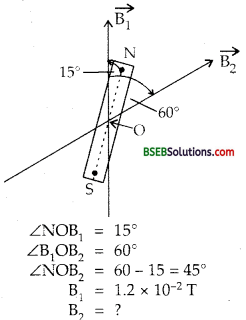Let θ1 and θ2 be the inclination of the dipole with $$\overrightarrow{\mathrm{B}_{\mathrm{1}}}$$ and $$\overrightarrow{\mathrm{B}_{\mathrm{2}}}$$ respectively.
θ1 = 15°, θ2 = 45°.
If τ1 and τ2 be the torques on the dipole due to $$\overrightarrow{\mathrm{B}_{\mathrm{1}}}$$ and $$\overrightarrow{\mathrm{B}_{\mathrm{2}}}$$ respectively, then
Using the relation, τ = MB sin θ, we get
τ1 = MB1 sin θ1 and
τ2= MB2 sin θ2
As the dipole is in equilibrium, the torques on the dipole due to $$\overrightarrow{\mathrm{B}_{\mathrm{1}}}$$ and $$\overrightarrow{\mathrm{B}_{\mathrm{2}}}$$ are equal and opposite . i.e.,
τ1 = τ2
or MB1 sin θ1, = MB2 sin θ2
or B2 = B1$$\frac{\sin \theta_{1}}{\sin \theta_{2}}$$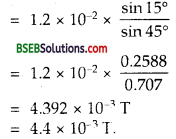Question 22.
A monoenergetic (18 keV) electron beam initially in the horizontal direction is subjected to a horizontal magnetic field of 0.40 G normal to the initial direction. Estimate the up or down deflection of the beam over a distance of 30 cm.
(me = 9.11 x 1031 kg, e = 1.60 x 1019 C).
Note : [Data in this exercise are so chosen that the answer will give you an idea of the effect of earth’s magnetic field on the motion of electron beam from the electron gun to the screen in a TV set.]
Here, Energy = E = 18 KeV = 18 x 1.6 x 10-16 J.
(∴ 1 KeV = 103ev = 103x 1.6x 1019J)
B = horizontal magnetic field = 0.40 G = 0.40 x 10-4 J.
m = 9.11 x 10-31 kg, e = 1.6 x 10-19C.
x = 30 cm = 0.30 m.
As the magnetic field is normal to the velocity, the charged particle
follows circular path in magnetic field. The centrepetal force $$\frac{\mathrm{mv}^{2}}{\mathrm{r}}$$ required for this purpose is provided by force on electron due to magnetic field, i.e., BeV
Here $$\frac{\mathrm{mv}^{2}}{\mathrm{r}}$$ = Be V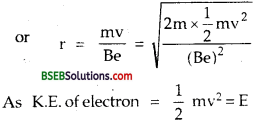Let y be the deflection at the end of the path, then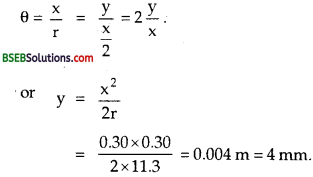Aliter:
Tire up and down deflection of the beam is equal to r (1 – cos θ)sin θ = $$\frac{0.30}{11.3}=\frac{3}{113}$$
= 0.02654
θ = sin (0.0264) 15
cos θ = 0.9997.
Up and down deflection of the beam = r (1 – cos θ)
= 11.3(1 – 0.9997)
= 11.3 x 0.0003
= 3.89 = 4 mm.Question 23.
A sample of paramagnetic salt contains atomic dipoles each of dipole moment 1.5 x 10-23 J T-1. The sample is placed under a homogeneous magnetic field of 0.64 T, and cooled to a temperature of 4.2 K. The degree of magnetic saturation achieved is equal to 15%. What is the total dipole moment of the sample for a magnetic field of 0.98 T and a temperature of 2.8 K? (Assume Curie’s law).
Here,no. of dipoles, n= 2 x 1024
Dipole moment of each dipole, M’ = 1.5 x 1023JT-1.
B1 = homogeneous magnetic field = 0.64 T.
T1 = initial temp, of sample = 4.2 K.
T2 = Final temp, of sample = 2.8 K.
M2 = total dipole moment of the sample at T2 = ?
B2 = Final magnetic field at T2 = 0.98 T.
Let M1 be the total initial dipole moment of the sample at magnetic.
Degree of saturation, D = 15%According to Curie’s law,
Xm = $$\frac {C}{T}$$ = $$\frac {I}{H}$$
I = C x $$\frac {H}{T}$$
But I ∝ magnetic moment, M and H ∝ magnetic induction, B
∴ From (i), Magnetic moment = $$\frac {C x B}{T}$$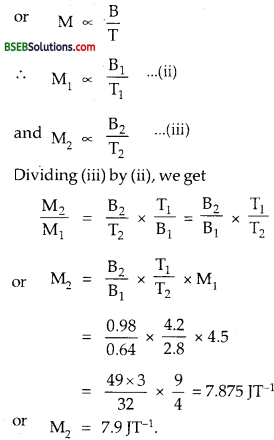Question 24.
A Rowland ring of mean radius 15 cm has 3500 turns of wire wound on a ferromagnetic core of relative permeability 800. What is the magnetic field B in the core for a magnetizing current of 1.2 A?
Here, a = mean radius of Rowland,
I = magnetising current = 1.2 A, ring = 15 cm = 0.15 m.
N = Total number of turns = 3500.
µr = relative permeability = 800
l = length over which the wire is wound.
= 2πa = 2π x 0.15 = 0.30 π.
If n be the number of turns per unit length, then
n = $$\frac{\mathrm{N}}{l}=\frac{3500}{0.30 \pi}=\frac{35 \times 10^{4}}{30 \pi}$$
∴ The magnetic field $$\overrightarrow{\mathrm{B}}$$ in the core is given by,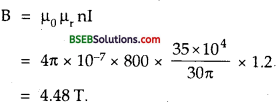Question 25.
The magnetic moment vectors ps and pr associated with the intrinsic spin angular momentum S and orbital angular momentum 1, respectively, of an electron are predicted by quantum theory (and verified experimentally to a high accuracy) to be given by:
$$\overrightarrow{\mathrm{µ}_{\mathrm{s}}}$$ = (e/m) $$\overrightarrow{\mathrm{S}}$$ , $$\overrightarrow{\mathrm{µ}_{\mathrm{l}}}$$ = (e/2m) $$\overrightarrow{\mathrm{l}}$$
Which of these relations is in accordance with the result expected classically? Outline the derivation of the classical result.
Out of these two relations, $$\overrightarrow{\mathrm{µ}_{\mathrm{s}}}$$ = $$-\frac{\mathrm{e}}{2 \mathrm{~m}}$$ $$\overrightarrow{\mathrm{l}}$$ is in accordance
with classical Physics and can be derived as follows :
We know that electrons revolving around the nucleus of an atom in circular orbits behave as tiny current loops having angular momentum
$$\overrightarrow{\mathrm{l}}$$ given in magnitude as :
$$\overrightarrow{\mathrm{l}}$$ = mvr …….(i)
where m = mass of an electron
v = its orbital velocity
r = radius of the circular orbit.
or v r = $$\frac {l}{m}$$ ……(ii)
$$\overrightarrow{\mathrm{l}}$$ acts along the normal to the plane of the orbit in upward direction.
The orbital motion of electron is taken as equivalent to the flow of conventional current I given by
I = $$\frac{e}{T}=\frac{e}{\left(\frac{2 \pi r}{v}\right)}=\frac{e v}{2 \pi r}$$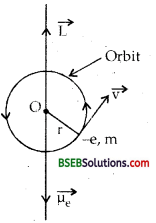∴ magnetic moment µr of the current loop due to orbital motion is given bywhere – ve sign shows that the electron is negatively charged. The eqn. (iii) shows that pe and $$\overrightarrow{\mathrm{l}}$$ are opposite to each other i.e., antiparallel and both being normal to the plane of the orbit as shown in the figure :
∴ $$\overrightarrow{\mathrm{µ}_{\mathrm{l}}}$$ = $$-\frac{\mathrm{e}}{2 \mathrm{~m}} \cdot \vec{l}$$

$$\frac{\mu_{\mathrm{s}}}{\mathrm{S}}$$ is contrast to $$\frac{\mu_{l}}{\vec{l}}$$ is $$\frac{\mathrm{e}}{\mathrm{m}}$$ i.e., twice the classically expected value. This latter result is an outstanding consequence of modern quantum theory and cannot be obtained classically.### Bihar Board Class 12 Physics Chapter 5 Magnetism and Matter Additional Important Questions and Answers

Question 1.
Define a magnet.
It is defined as a substance which can attract magnetic materials like iron etc. and rests in north-south direction when suspended freely. It has
two equal and opposite magnetic poles separated by a certain distance.

Question 2.
What is a sure test of magnetism? Name a natural magnet and give its chemical formula.
Repulsion is the sure test of magnetism. Magnetite is a natural magnet having chemical formula Fe304.

Question 3.
Define induce magnetism.
It is defined as the magnetism attained because of another magnet without physical contact.

Question 4.
Define pole strength of a magnet. The two poles of a magnet equally strong?
It is defined as the ability of a magnet to attract small pieces of magnetic material ike iron, nickel etc. by its poles.
Yes, the two poles of a magnet are equally strong.

Question 5.
Define the following:
(a) Terrestrial magnetism.
(b) Geographic meridian.
(c) Magnetic meridian.
(a) It is defined as the study of magnetic field of earth.
(b) Geographic meridian :It is defined as the vertical plane passing through the geographic axis i.e., geographical N-S line.
(c) It is defined as the vertical plane passing through the magnetic
axis of the earth. .

Question 6.
Define geomagnetic equator.
It is defined as the great circle on earth’s surface whose plane is perpendicular to the magnetic axis.

Question 7.
What do you mean by the directive property of a magnetic dipole?
It is that properly of a freely suspended magnet due to which it always aligns itself along the N-S line.

Question 8.
What is the basic difference between magnetic lines of force and electric lines of force?
The magnetic lines of force are always closed loops. As isolated magnetic poles do not exist, they always run from N-pole to south pole
outside the magnet and S-pole to N-pole inside the magnet. On the otherhand, the electric lines of force originate from positive charge, and terminate on the negative charge and are thus discontinuous curves.

Question 9.
If a bar magnet is cut into two equal parts parallel to its length, then what happens to its dipolemoment?
Let m, 21 be the pole strength and the dipole length of the magnet. If M be the magnetic moment of the magnet, then
M = m. 2l
when it is cut into two equal pieces parallel to its length, then each
piece will be a magnet having length 2l and pole strength $$\frac {m}{2}$$
Thus the magnetic moment of each piece will be $$\frac {M}{2}$$. (2l) = $$\frac {1}{2}$$ . [m = 2l] = $$\frac {M}{2}$$
Hence the magnetic moment will become half of that of the original magnet.

Question 10.
Define magnetic permeability.
It is defined as the degree to which the magnetic lines of force can penetrate a substance. It is denoted by g.

Question 11.
Differentiate between magnetic intensity and intensity of magnetisation.
Magnetic Intensity – It is defined as the degree to which a magnetic field line can magnetise a substance. It is denoted by H.
Intensity of Magnetisation – It is defined as the degree to which a substance is magnetised on placing it in a magnetic field. It is denoted by I.Question 12.
What happens to the dipole moment of a bar magnet when it is cut into two equal pieces transverse to its length.
Each piece will be a magnet having pole strength m and length / if its original parameters were m and 2l. So magnetic moment of
each piece will be m. l = $$\frac {M}{2}$$ , where M = m. 2l. Thus the magnetic moment reduces to half value as compared to its original value.

Question 13.
Explain why ordinary piece of iron don’t behave as a magnet ?
The molecular magnets in ah ordinary piece of iron are randomly oriented and form closed chains, thus cancel the effect of each other, so it does not behave as a magnet.

Question 14.
(a) Define a magnetic dipole.
(b) What is a natural fundamental dipole? Explain.
(a) It is defined as a pair of magnetic poles of equal and opposite pole strength separated by a small distance.
(b) Electron is a natural fundamental dipole. The electrons revolving in an atom behave as tiny current loops which give rise to the magnetism.

Question 15.
(a) What will happen to a magnetic core placed in a solenoid carrying no current?
(b) How can you determine the polarity of a solenoid?
(a) It will not be magnetised.
(b) Polarity of a solenoid can be determined with the help of clock rule. Anticlockwise current in a face of the solenoid gives north polarity to it and clockwise current gives S-polarity.

Question 16.
When does a magnetic dipole possess:
(a) maximum potential energy.
(b) minimum potential energy inside a magnetic field?
A magnetic dipole possesses :
(a) maximum potential energy in a magnetic field when the dipole is antiparallel to $$\overrightarrow{\mathrm{B}}$$ i.e., $$\overrightarrow{\mathrm{M}}$$ and $$\overrightarrow{\mathrm{B}}$$ are opposite (θ = 180°).
(b) minimum energy when it aligned with $$\overrightarrow{\mathrm{B}}$$ i.e.,θ = 0. –

Question 17.
Define magnetic elements. Name them.
They are defined as the physical quantities which determine the intensity of earth’s total magnetic field completely i.e.; both in magnitude and direction. Their names are: (i) Magnetic declination, (ii) dip or magnetic inclination, (iii) Horizontal Component of earth’s magnetic field. .

Question 18.
Define Bohr magneton and derive its value.
It is defined as the magnetic dipole moment associated with an atom due to orbital motion of an electron in the first orbit of hydrogen atom. It is denoted by μB.Question 19.
Define neutral point in the magnetic field of a bar magnet.
It is defined as that point where the field due to the magnet is . completely neutralised by the horizontal component of earth’s magnetic
field i.e., Bmag = BH at the neutral point.

Question 20.
What is the direction of horizontal component of earth’s magnetic field above the surface of earth?
The direction of horizontal component of earth’s magnetic field is from geographic south to geographic north above the surface of earth.Question 21.
How will a magnet suspended freely at equator and pole orient itself?
A magnet suspended freely at equator will orient itself parallel to the surface of earth while the magnet suspended freely at pole will stand vertically.

Question 22.
How does dip angle vary as one moves from magnetic equator to the pole?
The dip angle varies from 0° to 90° as one moves from magnetic equator to the pole.

Question 23.
In what direction would a compass needle align if taken to geographical (i) north and (ii) south pole?
A compass needle would align under the influence of vertical component because the horizontal component of earth’s magnetic field is zero at both the geographical north and south poles.

Question 24.
Define declination at a place. How does it help in navigation?
It is defined as the angle between the geographic and magnetic meridian at that place. By knowing the declination, the ship can be steered in the required direction, so as to reach the destination.

Question 25.
Define angle of dip at a place. What is its value in Britain as compared to India?
It is defined as the angle made by the direction of the earth’s total magnetic field with the horizontal direction. Britain is close to the magnetic north pole, so the angle of dip is greater in Britain than in India. Dip angle is about 70° in Britain and about 18° at a place in southern India which is $$\frac{1^{\text {th }}}{4}$$as in Britain.

Question 26.
A magnetic needle is placed on a cork floating on a still lake in the northern hemisphere. Does this needle together with the cork move towards the north of the lake?
The magnetic needle does not experience any net force and hence it along with the cork will not move towards the north of the lake and will come to rest along N-S direction. But if the needle is inclined to the magnetic meridian, a torque will act on the needle and after a few oscillations, it will set itself along the magnetic meridian.

Question 27.
The earth’s core is known to contain iron, yet geologists don’t regard this as a source of earth’s magnetism. Why?
The molten iron is above its Curie temperature, so it cannot retain magnetism. Hence it cannot remain as a magnet.

Question 28.
Define magnetic flux and magnetic flux density.
Magnetic flux—It is defined as the total number of magnetic lines of force passing normally through the unit area of a substance. It is denoted by ø It is also commonly known as magnetic field or strength of magnetic field.

Question 29.
Compare the magnetic fields of a solenoid and a bar magnet.
The magnetic field inside a solenoid is stronger than inside a bar magnet.

Question 30.
State Curie’s law in magnetism.
It states that the magnetic susceptibility of a material is inversely proportional to its absolute temperature i.e., mathematically Where C is proportionality constant known as Curie constant.
Xm ∝ $$\frac {1}{T}$$
or Xm = $$\frac {C}{T}$$
Where C is proportionality constant known as Curie constant

Question 31.
Give the value of Curie temperature for iron, cobalt and nickel.
The Curie temperatures for iron, cobalt and nickel are 1000 K, 1375 K and about 625 K respectively.

Question 32.
Which material have permeability more than one (1) ?
Paramagnetic and Ferromagnetic materials have permeability more than one.

Question 33.
State two methods of how to demagnetise a bar magnet?
A bar magnet can be demagnetised by :
(i) hammering it repeatedly.
(ii) heating it.

Question 34.
How does the intensity of a paramagnetic material vary with temperature?
Paramagnetic materials obey Curie Law of magnetism and their intensity decrease with increase in temperature.
i.e I ∝ $$\frac {1}{T}$$

Question 35.
Where the neutral points lie when the magnet is pie ced with its N-pole towards north of earth? Write the expression for its magnetic moment.
The neutral points lie on the equitorial line of its bar magnet when its N-pole points towards N-of earth.$$\frac{4 \pi}{\mu_{0}}$$ r3 BH for a short bar magnet.

Question 36.
Where the neutral points lie when S-pole of the magnet points towards the N-of earth? Write the expression for its magnetic moment.
The neutral points lie on the axial line of the bar magnet when S-pole of the magnet points towards N-of earth.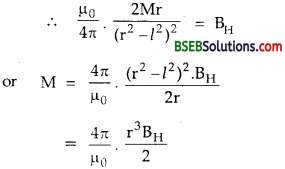for a short bar magnet.

Question 37.
Two magnets are placed one over the other in the stirrup of vibration magnetometer. When will their time period be maximum?
The time period of vibration of the combination will be maxium when the north pole of one magnet lies over the south pole of other and vice-versa.Question 38.
Name the states of matter which normally don’t show ferromagnetism?
Liquid and gases are the states of matter which normally don’t show ferromagnetism.

Question 39.
How will you judge as to which of the two given similar magnets is stronger, without using a third magnet?
The magnet which vibrates faster will be stronger as it will have lesser time period. We know that the time period of vibration is given by :
T = 2π $$\sqrt{\frac{I}{M B_{H}}}$$
Where I = M.I. of the magnet.
M = its magnetic moment m (pole strength)
BH = horizontal component of earth’s magnetic field.
T ∝ $$\frac{1}{\sqrt{\mathrm{M}}}$$ as I, BH and magnetic lengths of magnets are same.
Higher the pole strength lesser will be the time period.

Question 40.
Why a tangent galvanometer fails to work at magnetic north pole of earth?
It fails to work because horizontal component of earth’s magnetic field BH at the poles of earth is zero.

Question 41.
How does the intensity of magnetisation of a paramagnetic material vary with increasing applied magnetic field?
It directly increases with the increase in applied magnetic field.

Question 42.
What happen when a diamagnetic material is placed in a varying magnetic field?
It tends to move from the stronger to the weaker part of the magnetic field.

Question 43.
Why the electromagnets are made of soft iron?
The electromagnets are made of soft iron because coercivity of soft iron is small.

Question 44.
Why do magnetic lines of force prefer to pass through iron than air?
The magnetic lines of force prefer to pass through iron because the permeability of soft iron is much greater than that of air.

Question 45.
Magnetisation and de-magnetisation of soft iron is easier more difficult as compared to steel. Why?
Magnetisation and demagnetisation of soft iron is easier as compared to steel because coercivity of soft iron is smaller than that of steel.

Question 46.
What is the susceptibility and permeability of a-perfectly diamagnetic substance? Why.
The susceptibility (Xm) and permeability (μ) of a perfectly diamagnetic substance are -1 and zero respectively.
We know that B = μ0 (H + I).
B = 0 for perfectly diamagnetic material.
∴ H + I = 0 or l = -HQuestion 47.
Define reduction factor.
It is defined as the current required to produce a deflection of 45° in a tangent galvanometer.Question 1.
What are properties of a bar magnet?
The following are the main properties of a bar magnet:

• Property of attraction : The magnet attracts small pieces of magnetic substances.
• Directive properly or property of alignment – A freely suspended magnet always aligns itself along geographical N-S line.
• Like poles repel and unlike poles always attract each other.
• Property of pairs – Magnetic poles always exist in pairs and monopoles never exist.
• Repulsion is the surest test for distinguishing between a magnet and a piece of iron.
• Property of induction – When a piece of ordinary magnetic material is brought near a bar magnet, it acquires the property of a magnet without any actual contact.

Question 2.
State various properties of magnetic lines of forces.
The following are the important properties of magnetic lines of forces :

• They always from closed and continuous loops travelling from N to S. outside and S to N. inside the magnet.
• The tangent to the field lines at any point gives the direction of magnetic field at that point.
• No two magnetic lines of force intersect each other.
• The lines of force are not real but they represent a magnetic . field which is real.
• Widely spaced lines of force represent weak magnetic field and closely spaced lines represent strong magnetic field.

Question 3.
Derive the relation between permeability and susceptibility.
We know that the total magnetic flux density $$\overrightarrow{\mathrm{B}}$$ in a material is the sum of the magnetic flux density in vacuum ($$\overline{B_{0}}$$) produced by magnetising force and the magnetic flux density due to magnetisation of the material Bme. i.e.
B = B0 + Bm …..(i)
Also we know that
B0 ∝ H or B0 = μ0H …..(ii)
and Bm ∝ I or Bm = μ0I …..(iii)
Where H and I are magnetic intensity and intenstiy of magnetisation respectively.
∴ from (i), (ii) and (iii), we get
B =μ0H + μ0I = μ0(H + I) …(iv)
Also we know that, B = μH
Xm = $$\frac {1}{H}$$ or I = Xm H ….(v)
from (iv) and (v), we get
μH = μ0 (H + XmH)
or μ = μ0 (1 + Xm)
or $$\frac{\mu}{\mu_{0}}$$ = 1 + Xm
μr = 1 + Xm …….(iv)
where μr = $$\frac{\mu}{\mu_{0}}$$ is called relative permeabìhty of the medium. Equ. (vi) is the required relation.Question 4.
State Coulomb’s law of magnetic force.
It states that the force between two magnetic poles is always directly proportional to the product of their pole strengths and inversely proportional to the square of the distance between theìr centres i.e.
Mathematically,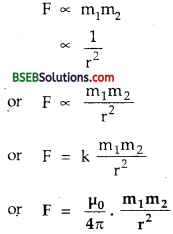where m1, m2 are the pole strengths of the two magnetic poles.
r = distance between them.
K = $$\frac{\mu_{0}}{4 \pi}$$ is the magnetic force constant having value 10-7
WbA-1 m-1 in S.L system. μ0 = absolute permeability of free space.

Question 5.
Derive the exsion for the torque ona bar magnet placed in a uniform magnetic field.
Let 21 be the length of a bar magnet NS having pole strength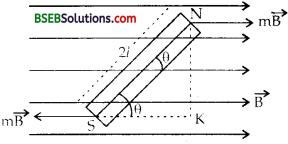Let it be placed in a uniform magnetic field $$\overrightarrow{\mathrm{B}}$$.
Let O be the angle made by the magnet with $$\overrightarrow{\mathrm{B}}$$ .
If $$\overrightarrow{\mathrm{M}}$$ be the magnetic dipole moment of the magnet,
Then $$\overrightarrow{\mathrm{M}}$$ =m. $$\overrightarrow{\mathrm{2l}}$$ or M = m 2l ……(1)
Force on each pole = m $$\overrightarrow{\mathrm{B}}$$ .
N and S poles expereicnce this force along and opposite to the field $$\overrightarrow{\mathrm{B}}$$
these two equal, parallel at’d opposite forces constitute a torque ($$\overrightarrow{\mathrm{τ}}$$) given by
τ = either force x perpendicular distance between the two forces
= mB x KN
Now in right angled ∆ NKS,
KN = NS sin θ = 2 l sin θ
.. From (2) and (3), we get
τ = mB x 2l sin θ
= m.2l. B sin θ
τ = MB sin θ
or in vector form,
$$\overrightarrow{\mathrm{τ}}$$ = $$\overrightarrow{\mathrm{M}}$$ x $$\overrightarrow{\mathrm{B}}$$Question 6.
Prove that U = – $$\overrightarrow{\mathrm{M}}$$ • $$\overrightarrow{\mathrm{B}}$$ for a magnetic dipole placed in a uniform magnetic field.
We know the magnitude of the torque acting on a dipole placed in a uniform field $$\overrightarrow{\mathrm{B}}$$ is given by
τ = MB sin θ …..(1)
This torque tends to align the dipole along the direction of $$\overrightarrow{\mathrm{B}}$$
Let d W be the work done in rotating the magnet by small angle dθ,
dW = τ d0 = MB sin θ dθ ………(2)
If W be the total work done in rotating the dipole from initial position θ1to final position θ2, then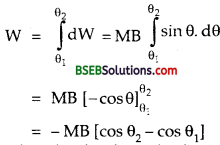This work done is stored in the dipole in the form of potential energy (= U) i.e.,
U = -MB (cos θ2 – cos θ1).
If the magnet be rotated from initial perpendicular position to a position making an angle 0 i.e., if θ1 = 90°, θ2, = θ, then
U = – MB (cos θ – cos 90)
= – MB (cos θ – 0)
= – MB cos θ
or U = – $$\overrightarrow{\mathrm{M}}$$ • $$\overrightarrow{\mathrm{B}}$$

Question 7.
Prove that a current loop behaves as a magnetic dipole.
Consider a circular coil carrying a current I in anticlockwise direction.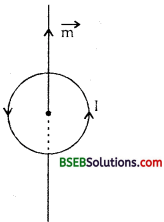It behaves as a magnetic dipole having dipole moment M which
(i) directly proportional to the current I in the loop. i.e.,
M ∝ I ……..(i)
(ii) directly proportional to the area A of the current loop i.e..,
M ∝ A …….(ii)
Combining (i) and (ii), we get
M ∝ IA
or M = KIA …..(iii)
Where K is the constant of proportionality. When I is measured in ampere, A in m2 and M in Am2; then K = 1.
∴ M = IA
The direction of dipole moment is perpendicular to the face area and along the axis of the loop according to right hand rule.

Question 8.
State Gauss’s law in magnetism. What are its consequences?
It states that the surface integral of the magnetic field $$\overrightarrow{\mathrm{B}}$$ over a closed surface S is always zero, i.e., mathematically
$$\oint_{S} \vec{B} \cdot \overrightarrow{d S}$$ = 0
Consequences of the law :
(i) Magnetic poles exist in unlike pairs of equal strengths.
(ii) If a number of magnetic field lines are leaving a closed surface, an equal number of field lines must also be entering the surface.
meaning..Question 9.
Prove that tan δ = $$\frac{B_{V}}{B_{H}}$$ where the symbols have their usual meaning.
Let $$\overrightarrow{\mathrm{B}}$$ be the earth’s total magnetic field at a place represented
δ = angle of dip.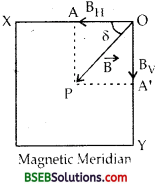Draw PA and PA’ perpendiculars to OX and OY respectively. Then OA and OA’ represent the horizontal component (BH) and vertical
component (Bυ) of $$\overrightarrow{\mathrm{B}}$$
∴ In rt. ∠d ∆ OAP,Hence proved.

Question 10.
State and prove tangent law of magnetism.
It states that if a small bar magnet is suspended in two mutually perpendicular uniform magnetic fields $$\overrightarrow{\mathrm{B}}$$ and $$\overrightarrow{\mathrm{B}_{\mathrm{H}}}$$ such that it comes
to rest making an angle θ with the direction of BH, then B = BH tan θ.Proof :
Let NS be a small bar magnet of length 2l having pole strength m.
θ = angle made by it with BH under equilibrium condition in the two mutually perpendicular fields B and BH.
If $$\overrightarrow{\mathrm{τ}_{\mathrm{1}}}$$ and $$\overrightarrow{\mathrm{τ}_{\mathrm{2}}}$$ be the torques on it due to BH and B respectively, then
τ1 = mBH x LN …….(i)
τ2 = mB x LS ……..(ii)
Under equilibrium condition
τ1 = τ2
or mBH x LN = mB x LS
or B = BH $$\frac {LN}{LS}$$ ……..(iii)
Now in rt. ∠d ∆ NSL,
tan θ = $$\frac {LN}{LS}$$ ………(iv)
From (iii) and (iv), we get
B = BH tan θ

Question 11.
Classify the magnetic materials into various categories and Explain.
The magnetic materials are classified into the following three categories:
(i) Diamagnetic – They are defined as the substances which when placed in a magnetic field are feebly magnetised in a direction opposite to that of the magnetising field.
(ii) Paramagnetic – They are defined as the materials which when placed in a magnetic field are feebly magnetised in the direction of the magnetising field.
(iii) Ferromagnetic – They are defined as the materials which when placed in a magnetic field are strongly magnetised in the direction of the magnetising field.

Question 12.
State the properties of Diamagnetic substances.

• They are feebly repelled by a magnet.
• A diamagnetic rod freely suspended inside a magntic field, slowly sets>itself at right angles to the direction of the external field.
• The magnetic lines of force don’t pass through them when placed in a magnetic field.
• The flux density ($$\overrightarrow{\mathrm{B}}$$) inside a diamagnetic material is less than in air.
• Permeability (μ) is always less than one. i.e., μ < 1.
•  Intensity of magnetisation (I) has small negative value.
• Susceptibility (Xm ) has a small negative value.
• They don’t obey Curie’s law.

Question 13.
State the properties of Paramagnetic substances.

• They are feebly attracted by a magnet.
• A paramagnetic rod freely suspended inside a magnetic field slowly sets itself along the direction of external magnetic field.
• Most of the magnetic lines of force pass through them when placed inside a magnetic field.
• The flux density inside a paramagnetic material is more than in air.
• ρ is more than one. i.e., μ > 1.
• Intensity of magnetisation (I) has a small positive value.
• Susceptibility has a small positive value i.e., Xm is + ve.
• They obey Curie’s law.

Question 14.
State various properties of Ferromagnetic materials.

• They are strongly attracted by a magnet.
• A freely suspended ferromagntic rod quickly sets itself alongi the direction of external magnetic field.
• All the magnetic lines of force prefer to pass through them when they are placed inside a magnetic field.
• The flux density inside such materials is much larger than in air i.e., B > > 1.
• μ is much more than unity.
• Intensity of magnetisation has larger positive value.
• Xm has larger positive value.
• They obey Curie’s law.

Question 15.
Define the following:
(a) Hysteresis
(b) Residual magnetism or remanence or retentivity
(c) Coercivity
(d) Hysteresis loss.
(a) It is defined as the lag of intensity of magnetisation behind the magnetising field during the process of magnetisation and diamagnetisation of a ferromagnetic material. It is shown in the figure. The curve ABCDEFA is known as hysteresis loop.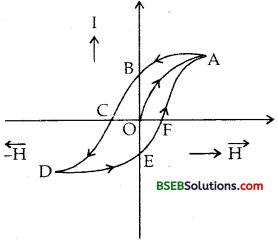(b) Retentivity – It is defined as the value of intensity of magnetisation of a material when the magnetising field is reduced to zero, e.g., OB represents retentivity of the material.
(c) Coercivity – It is defined as the value of the reverse magnetising field required to reduce the residual magnetism to zero.
e.g., OC represents coercivity of material.
(d) Hysteresis loss – It is defined as the energy loss per unit volume per cycle of magnetisation. It is equal to the area under I – H curve.Question 16.
Derive the expression for time period of vibration of a vibration magnetometer.
Let M be the magnetic moment of a bar magnet placed in a magnetic field BH making an angle θ,
If x be the restoring torque acting on it, then
τ = MBm sin θ ……(i)
If θ is small, then sin θ ≈ θ.
∴ τ = -M BHθ …..(ii)
Let a be the angular acceleration of the magnet having moment of inertia I about the axis of rotation, then
τ = Iα …….(iii)∴ from (ii) and (iii), we get
Iα = – MBHθ
or α = $$\left(\frac{\mathrm{MB}_{\mathrm{H}}}{\mathrm{I}}\right)$$ θ
Now an α ∝ θ and acts towards the mean position, so the motion of the magnet is S.H.M. having time period T given by
T = 2π $$\sqrt{\frac{\theta}{\propto}}$$
T = 2π$$\sqrt{\frac{\mathrm{I}}{\mathrm{MB}_{\mathrm{H}}}}$$

Question 17.
On what factors reduction factor of a tangent galvanometer depends? Write its expression.
It depends on :
(i) The no. of turns (n) in the coil.
(ii) Radius of the coil (r)
(iii) Horizontal component of earth’s magnetic field at the place. Its expression is given by :
K = $$\frac{\mathrm{B}_{\mathrm{H}}}{\mathrm{G}}=\frac{\mathrm{B}_{\mathrm{H}}}{\mu_{0} \mathrm{n} / 2 \mathrm{r}}=\frac{2 \mathrm{rB}_{\mathrm{H}}}{\mu_{0} \mathrm{n}}$$

Question 1.
Derive the expression for magnetic field due to a bar magnet on its:
(a) Axial line.
(b) Equitorial line.
(a) B on the axial line of a bar magnet Let M = m. 2l be the magnetic moment of the bar magnet of length 2l having pole strength m. Let r be the distance of the point P from the centre O of the bar magnet on
its axial line at which $$\overrightarrow{\mathrm{B}}$$ is to be calculated.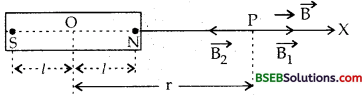If $$\overrightarrow{\mathrm{B}_{\mathrm{1}}}$$ and $$\overrightarrow{\mathrm{B}_{\mathrm{2}}}$$ be the magnetic fields at P due to N – and S – poles respectively, thenClearly $$\overrightarrow{\mathrm{B}_{\mathrm{1}}}$$ > $$\overrightarrow{\mathrm{B}_{\mathrm{2}}}$$
If $$\overrightarrow{\mathrm{B}}$$ be the total magnetic field at P due to the magnet, then$$\overrightarrow{\mathrm{B}}$$ = $$\overrightarrow{\mathrm{B}_{\mathrm{1}}}$$ + $$\overrightarrow{\mathrm{B}_{\mathrm{2}}}$$If bar magnet is short, then r> > l, so r2> > l2, hence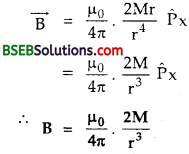(b) On the equitorial line – Let r be the distance o( the point P on the equitorial line OY of the bar magnet at which $$\overrightarrow{\mathrm{B}}$$ is to be calcula ted: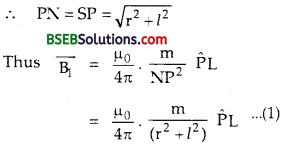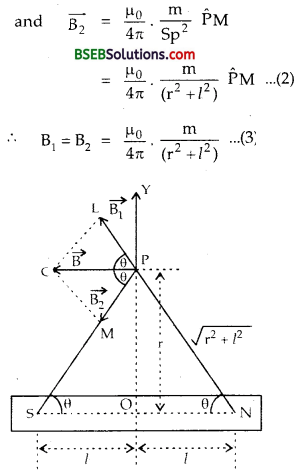If $$\overrightarrow{\mathrm{B}}$$ be the resultant field at P. ThenNow in rt.∠d ∆ OSP,cos θ = $$\frac {SO}{SP}$$ = $$\frac{l}{\sqrt{r^{2}+l^{2}}}$$ ……..(5)
∴ From (3), (4) and (5), we get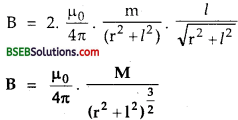and it acts along PC.
For a short bar magnet, 1 < < r,
B = $$\frac{\mu_{0}}{4 \pi} \cdot \frac{\mathbf{M}}{\mathbf{r}^{3}}$$

Numerical Problems

Question 1.
A magnetised wire of length 8 cm has a magnetic moment of 80 x 10-3 J T-1. It is then bent into a semicircular arc. What is the new magnetic moment in terms of π?
Here, magnetic length = 21 = 8 cm = 8 x 10-2 m.
M = magnetic moment = 80 x 10-3JT-1
If m be the pole strength, then using M = m. 2l, we get
m = $$\frac{\mathrm{M}}{2 l}=\frac{80 \times 10^{-3}}{8 \times 10^{-2}}$$ = 1.0 NT-1
When the wire is bent into a semicircular arc, the pole strength remains same but the separation between the poles is = 2r, r being the radius of the arc.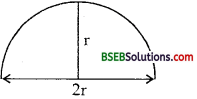Now 2l = semicircumference of the semicircle
= $$\frac{2 \pi r}{2}$$ = πr
∴ r = $$\frac{2l}{π}$$
Let M’ be the new magnetic moment.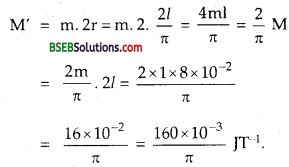Question 2.
A compass needle having magnetic moment 60 Am2 points towards geographical north at a certain place where the magnetic field is 40 g Wb nr2. It experiences a torque 1.2 x 10-3 Nm. Find the angle for torque?
Here, τ = 1.2 x 10-3 Nm.
M = Dipole moment = 60 Am2
B = flux density = 40 g Wb m-2 = 40 x 10-6Wb m-2.
θ = angle for torque = ?
Using the relation, τ = MB sin θ, we get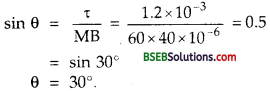Question 3.
Calculate susceptibility and permeability of a magnetic bar of 0.1 cm2 cross-sectional area having flux 2.41 x 10-5 Wb due to magnetic intensity of 3200 Am-1.
Here, A = Area of cross-section = 0.1 cm2 = 0.1 x 10-4 m2.
H = Magnetic intensity = 3200 Am-1.
Magnetic flux = ø = 2.41 x 10-5 Wb.
μ = permeability = ?
Xm = susceptibility = ?
We know that
μ = $$\frac{\mathrm{B}}{\mathrm{H}}=\frac{\frac{\phi}{\mathrm{A}}}{\mathrm{H}}$$ (∵ B = $$\frac{\phi}{\mathrm{A}}$$μ0 = absolute permeability of free space – 4K x 10-7TA-1m.
Using the relation,Question 4.
Find the magnetic moment of a rod of volume 105 mm3 and relative permeability 1000 under the effect of 2.5 A cm-1 magnetic intensity.
Here, V = Volume of rod = 103 mm3 = 105x (10-3 m)-3 = 105 x 10-9 m3 = 10-4 m3.
H = magnetic intensity = 2.5 A cm-1
= 2.5 $$\frac{\phi}{\mathrm{A}}$$ = 2.5 x 102 AM-1
μr = relative permeability = 1000.
M = magnetic moment = ?
We know that
I = $$\frac{\mathrm{M}}{\mathrm{V}}$$ or M = I x V ………(1)
l can be calcated from the relation,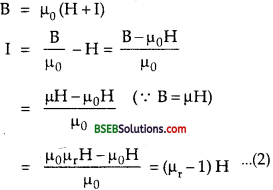From (1)and (2), we get
M = (μr – 1)H x V
= (1000 – 1) x 2.5 x 102 x 10-4
= 999 x 2.5 x 10-2
= 24.78 Am2
= 25 Am2.Question 5.
In a U-magnet distance between its two poles is 4 cm. Find the net force experienced by a unit pole placed in the middle of poles of the U-magnet if strength of its each pole is 4 NT-1.
Here, M = Pole strength of each pole
= 4 NT-1
R = distance between two poles = 4 cm = 4 x 10-2 m
Let r = distance of unit pole from each pole placed in betweehthem = $$\frac{\mathrm{R}}{\mathrm{2}}$$ = 2 x 10-2 m.
If F1 and F2 be the forces experienced by the unit pole, then according to Coulomb’s law of magnetism.Question 6.
An electron in an atom revolves around the nucleus in an orbit of radius 0.53 A°. Calculate the equivalent magnetic moment, if
the frequency of revolution of electron is 6.8 x i09 MHz.
Here, v = frequency of revolution of electron
= 6.8x 109 MHz = 6.8 x 109 x 106Hz.
= 6.8 x 1015 Hz.
r = radius orbit of the electron = 0.53 A°
= 0.53 x 10-10m.
M = magnetic moment of eletron =?
e = charge on an electron = 1.6 x 10-19 C.
T = time period of electron = $$\frac{\mathrm{1}}{\mathrm{v}}$$
If I = current equivalent to the motion of the electron, then
I = $$\frac{\mathrm{e}}{\mathrm{T}}$$ = ev = 1.6 x 10-19 x 6.8 x 1015 A.
A = Area of orbit = πr2 – π x (0.53 x 10-10)2
Using the relation, M = 1 A, we get
M = 1.6 x 10-19 x 6.8 x 10-15 x π x (0.53 x 1.0-10)2 = 9.6 x 10-24 Am2.

Question 7.
Two like magnetic poles of strength 25 Am and 64 Am are situated 1.0-m apart in air. At what point on the line joining the two poles, the magnetic field will be zero?
Here m1 = 25 Am, m2 = 64 Am, r = 1.0 m.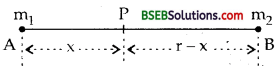Let P be the point at a distance x from nq at which the magnetic field is zero.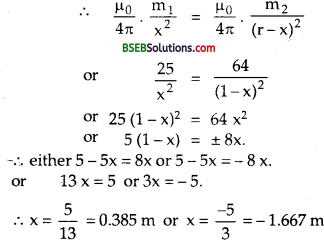∴ $$\overrightarrow{\mathrm{B}}$$ will be zero at a distance 0.385 m from the pole of 25 Am strength towards 64 Am or at a distance of 1.667 m from pole of 25 Am strength on the other side of it.

Question 8.
If δ1 and δ2 be the angles of dip in vertical planes at right angles to each other and 5 is the free angle of dip, then show that
cot2 δ = cot2 δ1 + cot2 δ2
Planes 1 and 2 are at right angles to each other.Let θ = angle made by plane 1 with the magnetic meridian.
∴ 90° – θ = angle made by plane 2 with the magnetic meridian.
The vertical components of earth’s magnetic field remain unaffected in two planes, the effective horizontal components of BH are BH COS θ and BH sin θ in the planes 1 and 2 respectively.
As δ is the true angle of dip.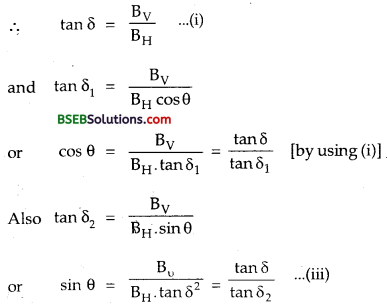Squaring and adding (ii) and (iii), we getQuestion 9.
The hysteresis loss for a sample of 12 kg is 300 Jm-3 cycle-1. ‘ Density of iron is 7500 kg m-3. Find the loss per hour at 50 cycles.
Here, U = energy loss/cycle/volume
= 300 Jm-3cycle-1.
p = density of iron = 7500 kg m-3.
M = mass of sample = 12 kg.
If V be the volume of the sample, then /
V = $$\frac{M}{\rho}=\frac{12}{7500} \mathrm{~m}^{3}=\frac{4}{2500} \mathrm{~m}^{3}$$
Let E be the energy loss per hour at 50 cycles = ?
∴ E = U x V x no. of cycles x 3600
= 300 x $$\frac {4}{2500}$$ x 50 x 36 x 4
= 3 x 800 x 36 = 3 x 28,800 J
= 86,400 = 8.64 x 104 J.Question 10.
Two bar magnets placed together in a vibration magnetometer takes 3s for 1 vibration. If one magnet is reversed the combination takes 4s for vibration. Find the ratio of their magnetic moments.
Here, T1 = 3s, T2 = 4s, $$\frac{\mathrm{M}_{1}}{\mathrm{M}_{2}}$$ = ?
Using the relation,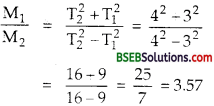Question 11.
A magnet vibrates 10 times in a minute. What is the number of vibrations if its pole strength is increased to four times?
Here, v1 = frequency of oscillations of the magnet = 10 vibr/ min.
Let m be the pole strength of the magnet of length 2l.
If M1 be the magnetic moment, then
M1 = m x 2l ,
Let m’ be the new pole strength, ∴ m’ = 4m.
If M2 be its magnetic moment, then
M2 = m’x 2l = 4 m x 2l = 4 M1
Let v2 be the frequency of vibration of the magnet = ?
We know that time period of oscillation is given by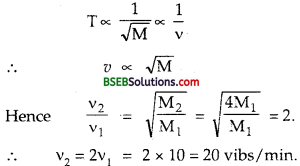Question 12.
A small magnet A makes 10 vibrations in 90 s in earth’s field. When another magnet B of short length is placed 0.1 m due south of the direction of earth’s field, the magnet A makes 10 vibrations in 45 s. Calculate the magnetic moment of magnet B. BH = 0.34 gause
Let I1 M1be the values of M,l. and magnetic moment of the magnet A.
If T1 be its time period in earth’s magnetic field, then
T1 = 2π$$\sqrt{\frac{\mathrm{I}}{\mathrm{M}_{1} \mathrm{~B}_{\mathrm{H}}}}$$ ……..(1)
Let T2 be the time period of magnet A when magnet B is placed due south of A. Now A lies on the axial line of the magnet BH thus if BH be the field in which now magnet A vibrates, then
BH = BH + Baxial ………(2)
where Baxial, is the magnetic field on the axial line of the magnet B at a point where A vibrates.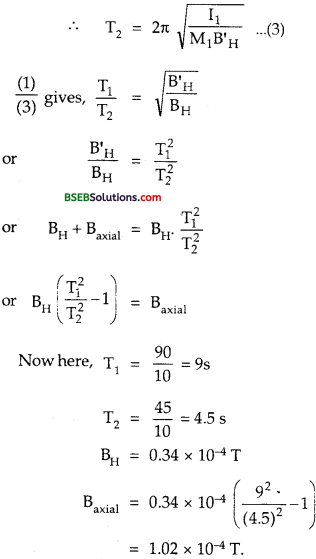Now Let M2 be the magnetic moment of the magnet B, then ’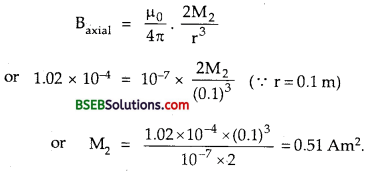Question 13.
The horizontal and vertical components of earth’s magnetic field at a place are 0.22T and 0.38 T respectively. Find the total intensity of earth’s magnetic field.
Here B11 = Horizontal component = 0.22 T
Bv = Vertical component = 0.38 T
B = earth’s total field = ?
Using the relation, B = $$\sqrt{B_{H}^{2}+B_{V}^{2}}$$ By – we get
B = $$\sqrt{(0.22)^{2}-(0.38)^{2}}$$ = $$\sqrt{0.1928}$$ = 0.44 T,Question 14.
A magnet 15 cm long with poles of strength 250 Am lies on the table. Find the magnitude of the magnetic intensity B at a point P 20 cm directly above the north pole of the magnet
Figure is shown here :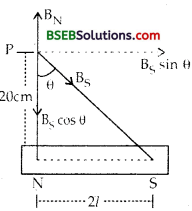Here, mN = ms= pole strength of each pole of the magnet = 250 Am.
r1= distance of point P from north pole = 20 cm = 0.20 m.
2l = 15m = 0.15 m
Let m = 1 be the pole strength of unit pole placed at P.
Let BN and Bs be the magnetic fields at P due to N and S poles respectively.
Using formula,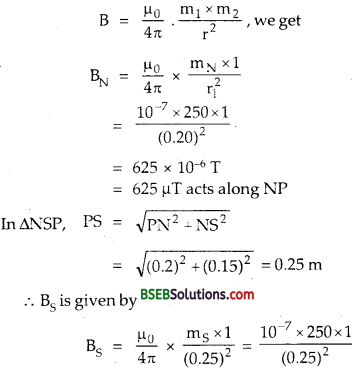= 400 μT a long PS.
Let θ = angle made by BS with PN.
The rectangular components of B are shown in the figure.∴ Net field along NP, BN = BN – BS cos θ
= 625 – 320 = 305 μT a long NP
If B be the resultant magnetic field at P, then
B = $$\sqrt{B_{1}^{2}+\left(B_{S} \sin \theta\right)^{2}}$$ = $$\sqrt{(305)^{2}+(240)^{2}}$$
= 390 μT.

Question 15.
A magnet makes 30 oscillations from at a place where BH = 1.8 x 10-5 T. At another place, it takes 1.6 s to complete one vibration. What is the value of earth’s horizontal component there?
Here, $$\mathrm{B}_{\mathrm{H}_{1}}$$ = 1.8 x 10-5T
υ1 = 30 oscillations/mm.
T1 = $$\frac{1}{v_{1}}=\frac{60}{30}$$ = 2s
T2 = 1.6 s
$$\mathrm{B}_{\mathrm{H}_{2}}$$ = ?

We know that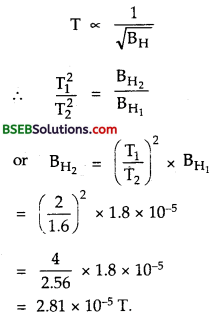Fill In The Blanks

Question 1.
A wire of length l metre carrying a current I ampere is bent into a circle. The magnitude of the magnetic moment in S.I. system is………
$$\frac{\mathrm{Il}^{2}}{4 \pi}$$

Question 2.
Lines joining the places having same angle of dip are called as ………
Isoclinic lines.

Question 3.
Angle between the magnetic meridian and the geographic meridian is called as ……….
Angle of declination.Question 4.
According to the modem theory of magnetism, every atom of a substance behaves just like a ……..
bar magnet.

Question 5.
The vertical component of earth’s magnetic field exists every where except at the ……….
Magnetic equator.

Question 6.
The electromagnets are made of soft iron because it has …….. and ……..
Low coercivity, high permeability.

Question 7.
Soft iron is preferred as the core of transformers due to its …….. and ……..
High retentivity, low coercivity, high permeability, low hystersis loss.

Question 8.
Lines joining the places having the same angle of declination are called …….
Isogonic lines.

Question 9.
In a hydrogen atom, the electron moves in an orbit of radius 0.5.0 A° making 1016 revolutions per second. The magnetic moment associated with the orbital motion of the electron is
1.26 x 10-23Am2.

Question 10.
The area under B-H or I-H loop gives the energy dissipated as heat and is known as hysteresis loss which is equal to
loss of energy per unit volume per cycle of magnetisation.

Question 11.
The Angle of dip is 0° at ………. and 90° at ………
Magnetic equator, magnetic poles.

Question 12.
Ampere/metre (Am-1) or Newton per Tesla per metre2 (NT-1m-2) is the S.I. unit of ………..
Magnetic intensity.

Question 13.
Ratio of force applied by the pole of a magnet on a unit north pole to the magnetic induction is called ……….
Pole strength (m) i.e.,
m = $$\frac {F}{B}$$

Question 14.
S.I. unit of magnetic permeability (p.) is …….
Wb A-1 m-1 or henry/metre (Hirr1).Question 15.
The potential energy of a magnetic dipole is minimum when it is ………. to the magnetic field and maximum when it is ……… to the magnetic field.
Parallel, Antiparallel.

Question 16.
The magnetic dipole is in the unstable equilibrium when $$\overrightarrow{\mathrm{M}}$$ and $$\overrightarrow{\mathrm{B}}$$ are ……….
Antiparallel, parallel.

Question 17.
The magnetic field inside a solenoid is than inside a bar magnet.